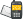It is currently 16 Jul 2019, 07:21### GMAT Club Daily Prep

#### Thank you for using the timer - this advanced tool can estimate your performance and suggest more practice questions. We have subscribed you to Daily Prep Questions via email.

Customized
for You

we will pick new questions that match your level based on your Timer History

Track

every week, we’ll send you an estimated GMAT score based on your performance

Practice
Pays

we will pick new questions that match your level based on your Timer History

#### Not interested in getting valuable practice questions and articles delivered to your email? No problem, unsubscribe here.# k is an integer for whichQuestion banks Downloads My Bookmarks Reviews Important topics
Author Message
TAGS:
FounderJoined: 18 Apr 2015
Posts: 7322
Followers: 122

Kudos [?]: 1430 , given: 6569

k is an integer for which [#permalink]
Expert's post00:00

Question Stats:58% (00:49) correct41% (00:55) wrongbased on 36 sessions

k is an integer for which $$\frac{1}{2^{(1-k)}} < \frac{1}{8}$$

 Quantity A Quantity B $$k$$ $$-2$$

A) Quantity A is greater.
B) Quantity B is greater.
C) The two quantities are equal.
D) The relationship cannot be determined from the information given.
[Reveal] Spoiler: OA

_________________GRE Prep Club LegendJoined: 07 Jun 2014
Posts: 4809
GRE 1: Q167 V156WE: Business Development (Energy and Utilities)
Followers: 122

Kudos [?]: 1958  , given: 397

Re: k is an integer for which [#permalink]
1
KUDOS
Expert's post
Explanation

We have the expression $$\frac{1}{2^{(1 - k)}}$$ and the fraction $$\frac{1}{8}$$.

Rewriting $$\frac{1}{8}$$ as $$\frac{1}{2^3}$$.

So if 1 -k > 3 (for example 1- k = 4) then the inequality $$\frac{1}{2^{(1 - k)}} < \frac{1}{8}$$.

So $$1 -k > 3$$ or $$-k > 2$$ or $$k <-2$$.

Thus Quantity B is greater.
_________________

Sandy
If you found this post useful, please let me know by pressing the Kudos Button

Try our free Online GRE Test

FounderJoined: 18 Apr 2015
Posts: 7322
Followers: 122

Kudos [?]: 1430 , given: 6569

Re: k is an integer [#permalink]
Expert's post
Explanation

the most common strategy here is testing number. Picking a number and substitute to k and see what happens. This is true but is time-consuming.

The best way to attack the question is to manipulate the stem

$$2^{1-k} > 8$$

$$2^{1-k} > 2^3$$

$$1-k > 3$$

$$-k > 2$$

$$k < -2$$ which means that B is greater. Hence, B is the right answer
_________________GRE InstructorJoined: 10 Apr 2015
Posts: 2144
Followers: 62

Kudos [?]: 1956  , given: 18

Re: k is an integer [#permalink]
3
KUDOS
Expert's post
Carcass wrote:
K is an integer for which

$$\frac{1}{2^{1-k}} < \frac{1}{8}$$

 Quantity A Quantity B k -2

A) Quantity A is greater.
B) Quantity B is greater.
C) The two quantities are equal.
D) The relationship cannot be determined from the information given.

Given: 1/[2^(1-k)] < 1/8

Since 2^(1-k) is POSITIVE for all values of k, we can safely take 1/[2^(1-k)] < 1/8 and multiply both sides by [2^(1-k)]
When we do this, we get: 1 < [2^(1-k)]/8
Rewrite 8 as follows: 1 < [2^(1-k)]/[2^3]
Since we have the same base, we can apply the quotient law to get: 1 < 2^(1 - k - 3)
In other words, 2^0 < 2^(1 - k - 3)
From this, we can conclude that 0 < 1 - k - 3
Simplify: 0 < -2 - k
Add k to both sides to get: k < -2

We get:
Quantity A: k (which is some number that's LESS THAN -2)
Quantity B: -2

RELATED VIDEOS

_________________

Brent Hanneson – Creator of greenlighttestprep.comInternJoined: 19 Jan 2018
Posts: 38
Followers: 1

Kudos [?]: 7 , given: 13

Re: k is an integer for which [#permalink]
I think the explanation and the problem post have a different fraction here
GRE Prep Club LegendJoined: 07 Jun 2014
Posts: 4809
GRE 1: Q167 V156WE: Business Development (Energy and Utilities)
Followers: 122

Kudos [?]: 1958 , given: 397

Re: k is an integer for which [#permalink]
Expert's post
chgre wrote:
I think the explanation and the problem post have a different fraction here

It was a formatting error. Its fixed now.
_________________

Sandy
If you found this post useful, please let me know by pressing the Kudos Button

Try our free Online GRE Test

InternJoined: 28 Feb 2018
Posts: 30
Followers: 0

Kudos [?]: 2 , given: 0

Re: k is an integer for which [#permalink]
BManagerJoined: 22 Feb 2018
Posts: 163
Followers: 2

Kudos [?]: 115  , given: 22

Re: k is an integer for which [#permalink]
1
KUDOS
correct: B
1 / 2^K-1 < 1/8
8 < 2 ^ 1-K
2^3 < 2 ^ 1-K
3 < 1-K
K < -2
so B is bigger

_________________

InternJoined: 18 May 2019
Posts: 2
Followers: 0

Kudos [?]: 1 , given: 0

Re: k is an integer for which [#permalink]
I m missing something here the fraction 1/2^(1-k)< 1/2^3 why r we fliping the sign when we ignore the numerator 1?

Posted from my mobile deviceFounderJoined: 18 Apr 2015
Posts: 7322
Followers: 122

Kudos [?]: 1430 , given: 6569

Re: k is an integer for which [#permalink]
Expert's post
Rule of exponents

$$\frac{1}{2^{(1-k)}} < \frac{1}{8}$$

Now, you can cross multiply

$$8 < 2^{1-k}$$

$$2^3 < 2^{1-k}$$

which means that the exponents look as the following

$$3 < 1-k$$

$$3-1 < -k$$

$$2 < -k$$

$$-2 > k$$

OR

$$k < -2$$

$$B > A$$
_________________InternJoined: 18 May 2019
Posts: 2
Followers: 0

Kudos [?]: 1  , given: 0

Re: k is an integer for which [#permalink]
1
KUDOS
thank you so much..that helped.

Posted from my mobile deviceRe: k is an integer for which   [#permalink] 23 Jun 2019, 01:48
Display posts from previous: Sort by

# k is an integer for whichQuestion banks Downloads My Bookmarks Reviews Important topicsPowered by phpBB © phpBB Group Kindly note that the GRE® test is a registered trademark of the Educational Testing Service®, and this site has neither been reviewed nor endorsed by ETS®.